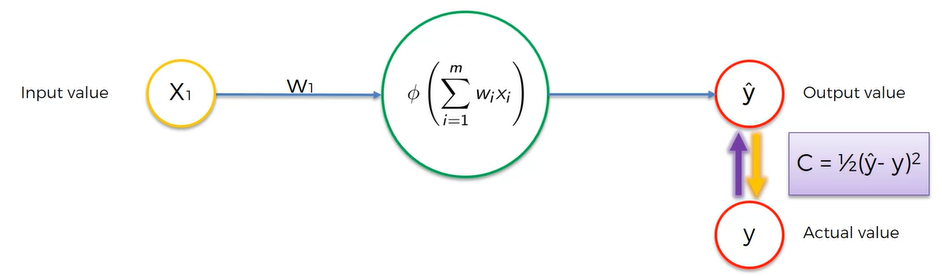Original article was published by Manik Soni on Artificial Intelligence on MediumGoals and Objectives :

2. What is an intuitive explanation of gradient descent?
3. What is the gradient descent algorithm?
4. What is the purpose of the use of gradient descent in machine learning
5. What is the science behind the gradient descent algorithm?

Prerequisites:

Before understanding the difference between gradient descent and stochastic gradient descent? You should first read the Fundamentals of Neural Network in Machine Learning.

So we have seen in the previous article on the fundamentals of neural networks and did the analysis that with backpropagation we are adjusting the weights. So, in order to adjust the weights in the neural network, we are using the concept of Gradient Descent.

Gradient Descent is basically the optimation done on the neural networks for minimizing the Cost Function.

So, basically, let us suppose there are thousands of weights that need to be adjusted and out of them which to choose so this Gradient Descent approach helps to do the optimization.

## What is the purpose of the use of gradient descent in machine learning?

So this is our already trained neural network.

But before the trained neural network, it looks like this:

That is a neural network having 25 weights and having a dataset with 1000 rows will make 1000²⁵ combinations.

So, Worlds fastest Super Computer can able to computer 93 PFLOPS(Petaflops) that is 93*10¹⁵ computations per second.

Now in order to consider all the combinations, it will take a more than a light-year to do the computation.

So we need to find an optimal way to do the computation. So, here comes Gradient Descent.

# What is an intuitive explanation of Gradient Descent?

So, we will look at our cost function and we see the faster way to do the optimization.

So, we start at some point.

Now, we will see the slope of our cost function, which will help to do the optimization.

Now if the slope is negative then we are going downhill.

Now, you need to go right that is downhill.

So this is the result

Now again do the same computation that is, calculate the slope and go left side.

Again repeat the process and do the calculation.

Now, this is the best way to find the minimum optimization with the help of Gradient Descent.

You can see within a few steps we came to an optimization.

In 2 Dimension you can see how Gradient Descent looks like?

In 3 Dimension you can see how Gradient Descent looks like?

Gradient Descent is good for the problems convex, that is the problems that are having Convex shape function.

But when the function is not in the convex shape that is hybrid.

So if we apply gradient descent on this function then it will do the optimization in local minima.

But the best optimization is this,

The limitation of Normal Gradient Descent is improved by Stochastic Gradient Descent.

Stochastic Gradient Descent may be defined as a modified gradient descent technique for doing the optimization globally.

So, if we consider a similar example that we have talked about in the Fundamentals of Neural Network in Machine Learning article.

So, we are doing the prediction of the exam result based on several attributes.

In order to adjust the weights, we are using 2 techniques:

1. Using normal Gradient Descent: In this method, we are adding all the rows and then reducing the cost function.

And then adjusting the weights until the cost function is minimized.

2. Using Stochastic Gradient Descent: In this method, we are adding the rows one by one.

and then we adjust the weights after adding the rows one by one.

again take the second row and adjust the weights.

We can visualize the difference:

1. In batch gradient descent we are getting the same result whereas, in stochastic gradient descent are getting random results in order to find global minima, not local minima.
2. Batch Gradient Descent is a faster method whereas, Stochastic Gradient Descent is a slower but accurate method.